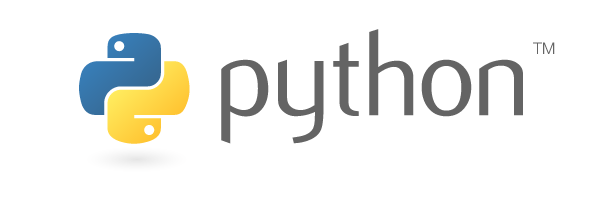# Basic Python Tutorial

This is a somewhat condensed tutorial for coding with Python, using the course material from our Robotics Club. The material regarding the Raspberry Pi itself will be outlined in another tutorial.

#### Introduction to PythonPython is a fun and easy programming language that has simple syntax. We can create the same code with fewer lines than in other languages like java. Python is great for beginners because it is easy to read and follow.

We can do many things with Python: create games, make phone apps, build robots, analyze data, and perform operations on the Raspberry Pi.

All Python coding can be done through an application called Nano, or a dedicated Python compiler if you happen to have one. However, this tutorial will be using Nano, so if you really want to follow along, make sure your computer both has Nano and can run Python programs.

#### First Program: Hello World!

What better place to start than the nigh-universal starting point for all programmers? To make Python print out “Hello world!”, we will use the print() function [eg. print(“Hello world!”)]. Any quoted text within the brackets of the function will be printed by Python when you run the program.

To create a Nano file, open your command prompt and type nano FileName.py (where FileName is what name you want to give your file, obviously). This should open a text editor where you can type characters into. Type in the code above before saving your code and exiting Nano using the instructions at the bottom of the editor (the sequence of keys pressed should be Ctrl-X –> Y –> Enter). Once you’re back in the command prompt, type in sudo python FileName.py to run your program. Your command prompt should show you the message “Hello world!”.

A few more tips and tricks for you before we move on:

• Python is extremely sensitive to indentation. If you see an indent in the tutorial, make sure you copy it! If there are more than one, you need the exact number of indents to make your code work.
• Use the Tab key for indents. If an indent is slightly off because you used the space bar, it won’t work.
• Use comments if you’re having trouble understanding your code. By putting a pound key (#) in front of a line, you can turn it into a comment that has no effect on your actual code. This lets you add notes and such to your code, making it easier to understand.

#### Variables and Types

In a programming language, variables are used to store information. In Python programming, you can name your variables whatever you want (within the variable naming rules), and have it represent a value. For example, if I wanted to keep track of the number of dogs that are in the park, I would call my variable , ‘num_of_dogs’, to represent the number of dogs in the park ( i.e if there were 5 dogs in the park, the value of the variable, ‘num_of_dogs’, would be ‘5’, coded as num_of_dogs = 5).

The variable naming rules are as follows:

• Can only contain letters, numbers and underscores
• They are case sensitive

There are multiple types of variables that can be used. Unlike many other programming languages, you do not have to declare the specific type when creating a variable. In fact, they can actually change types spontaneously!

 Variable Type Example value Integer 1, 2, -3, 0, 5, 467, -138, 483, 38, 472, etc.. Float 1.0, 3.14, -3.53, 1.0000, 1.00382, 3.8, etc… Character ‘A’, ‘b’, ‘@’, ‘ ‘, ‘5’, ‘P’, ‘9’, ‘\$’, etc… String (Group of Characters) ‘Hello’, ‘Hi’, ‘This is a String’, ‘huigrejbsdngasihdfbajg ierhj’, etc… Boolean True or False

It is also possible to change the value of a variable. Let’s say that another 3 dogs have entered the park. I would want to change the value of ‘num_of_dogs’ to 8, as 5 + 3 is equal to 8. To do this, you can just declare the same variable again (num_of_dogs = 8).

#### Input

Input allows the user to interact with their program while it’s running. We can also store inputs as variables and use them later. For example:

name = input(“What is your name?”)

This code will display “What is your name?” and wait for an input. When the user types something into the console, it will take this input and save it as the variable name.

#### Operators

Just like in math, we can use operators to compare two values. The list of valid operators are as follows

• (subtraction)
• * (multiplication)
• / (division)
• // (rounded division)
• % (modulus, divides the numbers and gets the remainder)

These are quite useful for having the computer perform calculations, such as getting the area of a shape after getting the dimensions from the user.

If/else and Conditional Statements

In addition to the operators above, we use conditional statements for comparing the relationship between two or more variables. In code, these are what we call if/else blocks – letting us ask the program yes/no questions.

To use it, you type if (condition): and then what you want the program to do if that condition is true. Keep in mind that you have to indent anything inside the if block. If you want to also do something if the condition is false, you put else: after the if block, on the same level of indentation as the original if statement, and put code into the else block.

Here is an example of how these could be used. This code gets a number from the user, and tells them if it’s odd or even.

num = int(input(‘What is your number?’))  #Asks the user for a number

if num % 2 == 0: #Checks if the number can be neatly divided by 2